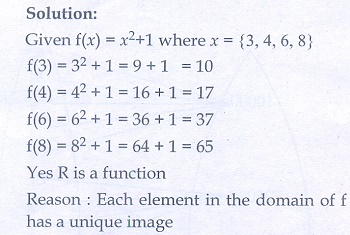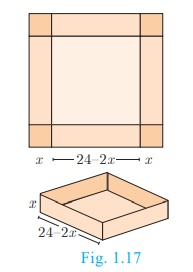Home | | Maths 10th Std | Exercise 1.3: Functions

# Exercise 1.3: Functions

Maths Book back answers and solution for Exercise questions - Mathematics : Function: Exercise Questions with Answer

### Exercise 1.3

1. Let  f  = {(x,y) | x,  N and = 2x} be a relation on N. Find the domain, co-domain and range. Is this relation a function?2. Let = {3, 4, 6, 8}. Determine whether the relation R = {(x(x)) |  X(x) = x2  + 1} is a function from to  N ?3. Given the function  x2 − 5+ 6 , evaluate

(i) f (-1)

(ii) f (2a)

(iii) f (2)

(iv) f (− 1)4. A  graph representing the function (x) is  given in Fig.1.16 it is clear that (9) = 2.(i) Find the following values of the function

(a) (0)

(b) (7)

(c) (2)

(d) (10)

(ii) For what value of is (x) = 1?

(iii) Describe the following (i) Domain (ii) Range

(iv) What is the image of 6 under ?5. Let (x) = 2x+5. If ≠ 0 then find6. A function is defined by (x) = 2– 3

(i) find [(0) + (1)] / 2.

(ii) find such that (x) = 0

(iii) find such that (x) = x

(iv) find such that (x) = (1 − x)7. An open box is to be made from a square piece of material, 24 cm on a side, by cutting equal squares from the corners and turning up the sides as shown (Fig.1.17). Express the volume of the box as a function of x.8. A function is defined by (x) = 3 − 2. Find such that (2 ) = ((x))29. A plane is flying at a speed of 500 km per hour. Express the distance travelled by the plane as function of time in hours.10. The data in the adjacent table depicts the length of a woman’s forehand and her corresponding height. Based on this data, a student finds a relationship between the height (y) and the forehand length(x) as ax , where aare constants.(i) Check if this relation is a function.

(i) Find and b.

(iii) Find the height of a woman whose forehand length is 40 cm.

(iv) Find the length of forehand of a woman if her height is 53.3 inches.1. {1, 2, 3, 4,...} , {1,2,3,4}, {2, 4, 6, 8,...} , yes.

2. yes

3.(i) 12 (ii) 4a2 − 10a + 6 (iii) 0 (iv) x2 − 7x + 12

4.(i) (a) 9 (b) 6 (c) 6 (d) 0

(ii) 9.5 (iii) (a) {x / 0 ≤ x ≤ 10, x  R} (b) {x / 0 ≤ x ≤ 9, x  R} (iv) 5

5. 2

6.(i) -2 (ii) 3/2 (iii) 3 (iv) 1/2

7. 4x3 − 96x2 + 576x

8. 1

9. 500t

10.(i) Yes (ii) 0.9,24.5 (iii) 60.5 inches (iv) 32 cms

Tags : Problem Questions with Answer, Solution , 10th Mathematics : UNIT 1 : Relation and Function
Study Material, Lecturing Notes, Assignment, Reference, Wiki description explanation, brief detail
10th Mathematics : UNIT 1 : Relation and Function : Exercise 1.3: Functions | Problem Questions with Answer, Solution